International Journal of Fluid Mechanics & Thermal Sciences
Volume 1, Issue 1, April 2015, Pages: 14-19

Evaluation of the Savonius Wind Rotor Performance for Different External Overlap Ratios

Zied Driss, Ali Damak, Mohamed Salah Abid

Laboratory of Electro-Mechanic Systems (LASEM), National School of Engineers of Sfax (ENIS), University of Sfax, Sfax, Tunisia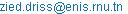(Z. Driss)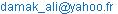(A. Damak)(M. S. Abid)

Zied Driss, Ali Damak, Mohamed Salah Abid. Evaluation of the Savonius Wind Rotor Performance for Different External Overlap Ratios. International Journal of Fluid Mechanics & Thermal Sciences. Vol. 1, No. 1, 2015, pp. 14-19. doi: 10.11648/j.ijfmts.20150101.13

Abstract: Experimental investigations were carried out to study the external overlap ratios effect on the performance of a vertical axis wind rotor of the Savonius type. For thus, an open wind tunnel has been designed and realized in Laboratory of Electro-Mechanic Systems at National School of Engineers of Sfax. This involved also the instrumentation and the necessary equipment for the global characterization of the Savonius wind rotor. Particularly, this research required the setting up of four mounting characterized by different external overlap ratios. The overall performance evaluation of the rotor has been based on the power and dynamic torque coefficients in function of the air velocity speed measured in the test vein of the wind tunnel.

Keywords: Savonius Rotor, External Overlap, Performance Evaluation, Wind Tunnel

1. Introduction

On the bases of these studies, evaluation of the Savonius wind rotor performance has been reported based on the average produced power and exerted torque in the case of different external overlap ratios.

2. Material and Method

2.1. Savonius Wind Rotor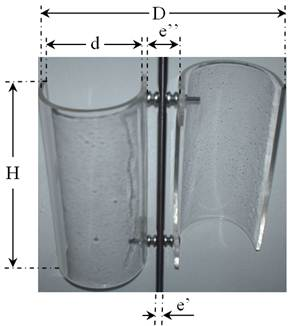D 100 mm H 200 mm e’ 10 mm e’’ variable D variable

Figure 1. Geometrical arrangement.

The rotor at hand is a Savonius wind rotor made on Plexiglas (Figure 1). It is constituted by two half-cylindrical buckets characterized by the diameter d=100 mm and the height H=200 mm. The buckets are collected on a common axis and are fixed within screws to make an angle equal to 180°. Indeed, the position between the two buckles is defined by the transversal distances designed by e’’. By introducing the shaft diameter e'=10 mm, we can define the external overlap ratio (e’’-e')/d. The study of the external overlap ratios effect on the Savonius wind rotor characteristics requires the e’’ distance change. In this investigation, we are interested in four mounting characterized by the external overlap ratios equal to (e’’-e')/d=0, (e’’-e')/d=0.1, (e’’-e')/d=0.2 and (e’’-e')/d=0.3 (Figure 2).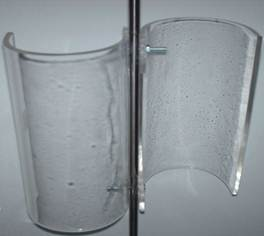(a) (e’’-e')/d=0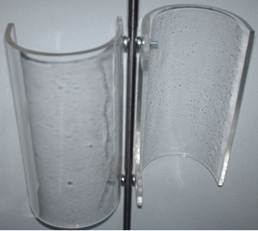(b) (e’’-e')/d=0.1(c) (e’’-e')/d=0.2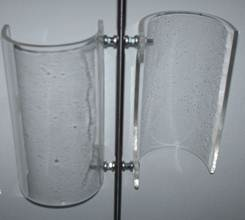(d) (e’’-e')/d=0.3

Figure 2. External overlaps.

2.2. Wind Tunnel and Experimental Method

Figure 3 shows the Savonius wind rotor placed in the middle of the wind tunnel test vein. The total length of the wind tunnel is 3857 mm. This tunnel is a fairly simple device and it consists of five compartments: a settling chamber, a collector, a test vein, a diffuser and a drive section. The test vein is of 400 mm of width, 800 mm of length and 400 mm of height. By changing the rotation frequency of the vacuum cleaner SV0081C5-1F type, the wind tunnel exit-air velocity was controlled. The entire tests have been conducted within a hot wire anemometry AM-4204 model to measure the air velocity. In the test vein, the maximum air velocity value is equal to 12.7 m.s-1. The rotational speed of the wind turbine rotor was measured with a digital tachometer CA-27 model. The dynamic torque exerted on the rotor shaft was measured with a DC generator which transforms the torque on its axis at an electrical current. For thus, the generator, coupled to the dynamometer RZR-2102 model, display simultaneously the shape speed and the dynamic torque. This dynamometer has been used to provide mechanical power to the generator which delivers an electric current in a resistive load.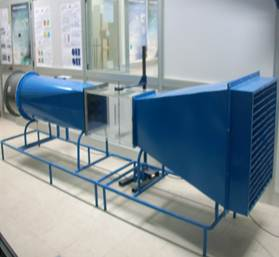Figure 3. Wind tunnel.

3. Experimental Results

3.1. Power Coefficient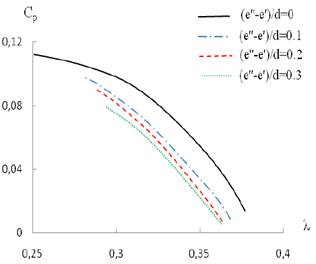(a) V=8.8 m.s-1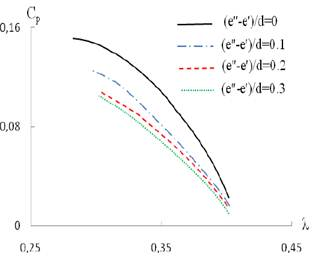(b) V=9.95 m.s-1(c) V=11.15 m.s-1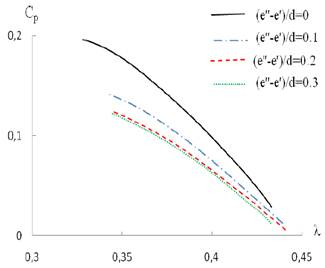(d) V=12.35 m.s-1

Figure 4. Variation of the power coefficient.

Figure 4 presents the variation of the power coefficient Cp depending on the specific speed λ of the Savonius wind rotor for different external overlaps equal to (e’’-e')/d=0, (e’’-e')/d=0.1, (e’’-e')/d=0.2 and (e’’-e')/d=0.3 and for different wind speed values equal to V=8.8 m.s-1, V=9.95 m.s-1, V=11.15 m.s-1 and V=12.25 m.s-1. In the case of the wind speed equal to V=8.8 m.s-1, we have calculated the corresponding values of Reynolds number for the different external overlaps. These values are equal to Re=103000, Re=108000, Re=115000 and Re=118000 respectively. The increase of the Reynolds number values is due to the increase of the Savonius wind rotor diameter when the external overlap value between the two buckets increases. According to these results, it has been noted that the gotten curves present a parabolic pace. Therefore, these results show that the external overlap value has a direct effect on the presentation of the power coefficient values. Globally, it has been noted that the Savonius wind rotor power coefficient reaches the most important values for the external overlap value equal to (e’’-e')/d=0. With the increase of the external overlap values, a progressive decrease of the power coefficient value has been observed. For the considered cases, it has been noted that the maximal value of the power coefficient is equal to Cp=0.11. It has been obtained with an external overlap value equal to (e’’-e')/d=0 and a specific speed equal to λ=0.25. With the increase of the external overlap value, it has been noted that the power coefficient values decrease. Particularly, for the external overlap value (e’’-e')/d=0.3 the power coefficient value becomes equal to Cp=0.06 for a specific speed equal to λ=0.3. For the other cases, these observations can be generalized.

3.2. Torque Coefficient

Figure 5 presents the variation of the torque coefficient CMd depending on the specific speed λ of the Savonius wind rotor for different external overlaps equal to (e’’-e')/d=0, (e’’-e')/d=0.1, (e’’-e')/d=0.2 and (e’’-e')/d=0.3 and for different wind speed values equal to V=8.8 m.s-1, V=9.95 m.s-1, V=11.15 m.s-1 and V=12.25 m.s-1. According to these results, it has been noted that the gotten curves present a parabolic pace. Therefore, these results show that the external overlap value has an effect on the presentation of the power coefficient values. Globally, it has been noted that the Savonius wind rotor torque coefficient reaches the most important values for the external overlap value equal to (e’’-e')/d=0. With the increase of the external overlap value, a progressive decrease of the torque coefficient value has been observed. In the case of the wind speed equal to V=8.8 m.s-1, it has been noted that the maximal value of the power coefficient is equal to CMd=0.45. It has been obtained with an external overlap value equal to (e’’-e')/d=0 and a specific speed equal to λ=0.26. With the increase of the external overlap value, it has been noted that the torque coefficient values decrease. Particularly, for an external overlap (e’’-e')/d=0.3, the torque coefficient value becomes equal to CMd=0.25 for a specific speed equal to λ=0.3. For the other cases, these observations can be generalized.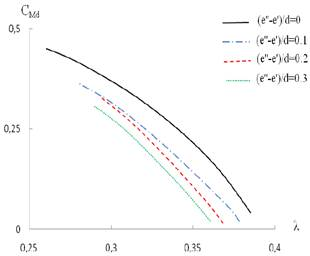(a) V=8.8 m.s-1(b) V=9.95 m.s-1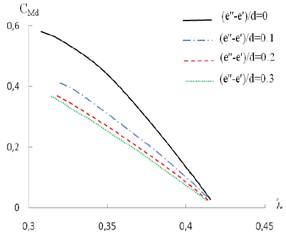(c) V=11.15 m.s-1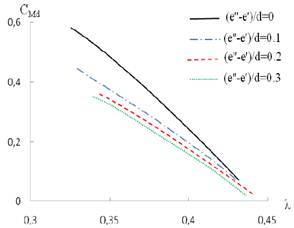(d) V=12.35 m.s-1

Figure 5. Variation of the torque coefficient.

4. Conclusion

In this paper, we have studied the global characteristics of the Savonius wind rotor with different external overlap. Particularly, we have reported evaluating the overall performance of the rotor based on the power and torque produced. The goal of this work is to optimize and to improve the experimental conditions of the Savonius wind rotor. In fact, the wind energy consist an inexhaustible and advantageous renewable energy regarding mass production of the electric energy. It answers to a long-term energizing strategy that is based on the principle of the lasting development and the protection of the environment.

In the future, we propose to change others geometrical parameters to improve the Savonius wind rotor performance.

References

1. D’Alessandro, V., Montelpare, S., Ricci, R., Secchiaroli, A., 2010, Unsteady Aerodynamics of a Savonius wind rotor: a new computational approach for the simulation of energy performance, Energy, 35, 3349-3363.
2. Altan, B.D., Atilgan, M., Özdamar, A., 2008, An experimental study on improvement of a Savonius rotor performance with curtaining, Experimental Thermal and Fluid Science, 32, 1673-1678.
3. Irabu, K., Roy, J.N., 2007, Characteristics of wind power on Savonius rotor using a guide-box tunnel, Experimental Thermal and Fluid Science, 32, 580-586.
4. Saha, U.K., Thotla, S., Maity, D., 2008, Optimum design configuration of Savonius rotor through wind tunnel experiments, Journal of Wind Engineering and Industrial Aerodynamics, 96, 1359-1375.
5. Fujisawa, N., 1996, Velocity measurements and numerical calculations of flow fields in and around Savonius rotors, Journal of Wind Engineering and Industrial Aerodynamics, 59, 39-50.
6. Al-Bahadly, I., 2009, Building a wind turbine for rural home, Energy for Sustainable Development, 13, 159-165.
7. Dobreva, I., Massouh, F., 2011, CFD and PIV investigation of unsteady flow through Savonius wind turbine, Energy Procedia, 6, 711-720.
8. Saha UK, M. Jaya Rajkumar, On the performance analysis of Savonius rotor with twisted blades, Renewable Energy 31 (2006) 1776-1788.
9. Saha, U.K., Thotla, S., Maity D., 2008, Optimum design configuration of Savonius rotor through wind tunnel experiments. Journal of Wind Engineering and Industrial Aerodynamics 96, 1359-1375.
10. Sabzevari, A., 1978, Power augmentation in a ducted Savonius rotor, Second international symposium on wind energy systems, 1-6.
11. Damak, A., Driss, Z., 2013, Abid M.S., Experimental investigation of helical Savonius rotor with a twist of 180°, Renewable Energy, 52, 136-142.
12. Driss, Z., Abid, M.S., 2012, Numerical and experimental study of an open circuit tunnel: aerodynamic characteristics, Science Academy Transactions on Renewable Energy Systems Engineering and Technology, 2 (1), 116-123.
13. Driss, Z., Mlayeh, O., Driss, D., Maaloul, M, Abid, M.S., 2014, Numerical simulation and experimental validation of the turbulent flow around a small incurved Savonius wind rotor, Energy, 74, 506-517.

 Contents 1. 2. 2.1. 2.2. 3. 3.1. 3.2. 4.
Article ToolsAbstractPDF(427K)